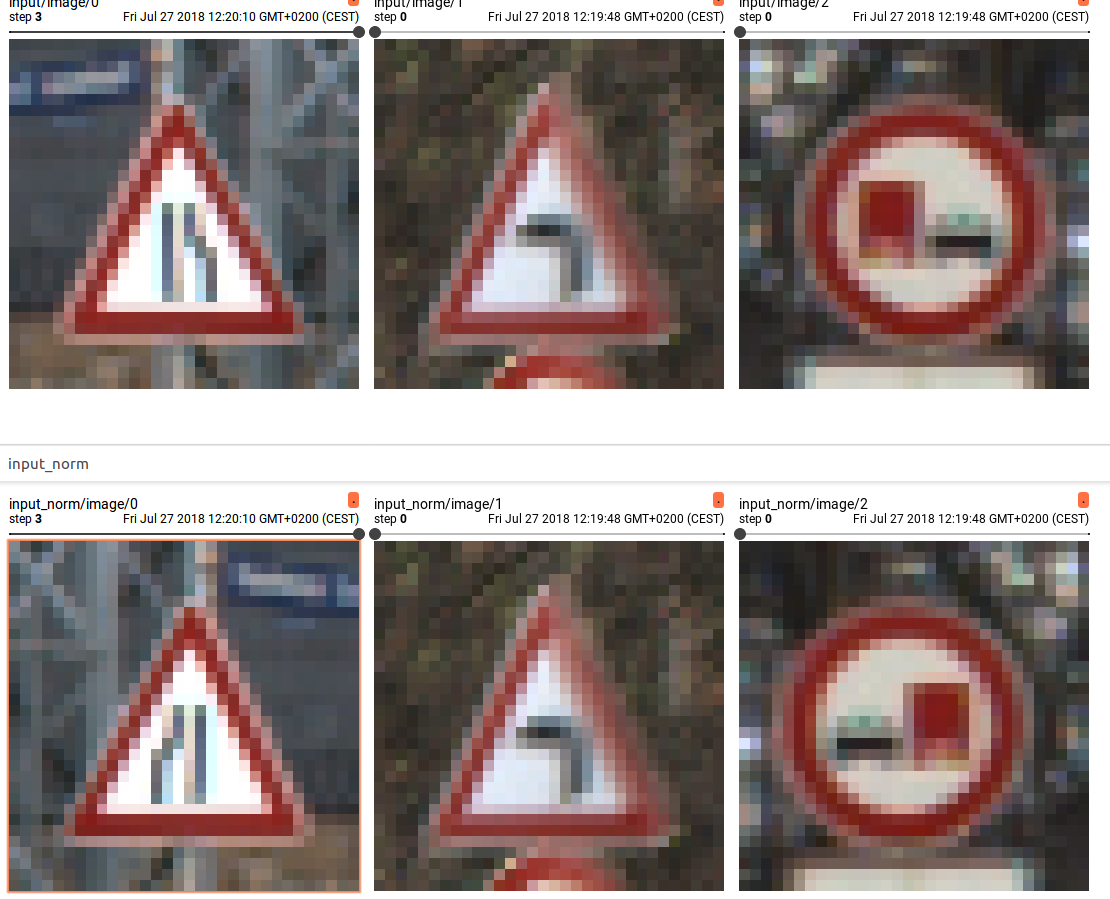# GTSRB

by Daniel Pollithy

## Classifying german traffic signs

Having reached something like a checkpoint in the cs231n lecture plan I decided to train a CNN to detect German street signs before going on with recurrent neural networks. Tensorflow will be employed and the network shall be deployed with tensorflow.js.

I am going to use the German Traffic Sign Benchmark whose leaderboard is currently lead by a team from the University of Seville where I spent an Erasmus semester.

The first step (as always) is to load the data. Fortunately the dataset came with a “getting started” script in python which I extended to scale the images to ndarrays IMAGE_SIZExIMAGE_SIZEx3.

``````% matplotlib inline

import numpy as np
import tensorflow as tf
import matplotlib.pyplot as plt
import csv
from skimage.transform import resize

# Cifar-10 was only 32x32 pixels. And it already took a vast amount
# of training. Therefore I am going to scale all images to:
IMAGE_SIZE = 32

# function for reading the images
# arguments: path to the traffic sign data, for example './GTSRB/Training'
# returns: list of images, list of corresponding labels
'''Reads traffic sign data for German Traffic Sign Recognition Benchmark.

Arguments: path to the traffic sign data, for example './GTSRB/Training'
Returns:   numpy array of shape(number_images, 32x32x3), list of corresponding labels'''
images = []
labels = [] # corresponding labels
# loop over all 43 classes
for c in range(0,43):
print('Class', c)
prefix = rootpath + '/' + format(c, '05d') + '/' # subdirectory for class
gtFile = open(prefix + 'GT-'+ format(c, '05d') + '.csv') # annotations file
# loop over all images in current annotations file
# until limit
class_limit = float('inf') if limit is None else float(limit)
class_counter = -1
class_counter += 1
if class_counter >= class_limit:
break

img_resized = resize(plt.imread(prefix + row), (IMAGE_SIZE, IMAGE_SIZE), anti_aliasing=False)
img_flat = img_resized.reshape(1, -1)
images.append(img_flat)
# images.append(img_resized) # the 1th column is the filename
labels.append(row) # the 8th column is the label
gtFile.close()
return np.vstack(images), np.asarray(labels).astype(np.uint8)

plt.imshow(trainImages.reshape((IMAGE_SIZE,IMAGE_SIZE,3)))
plt.show()
``````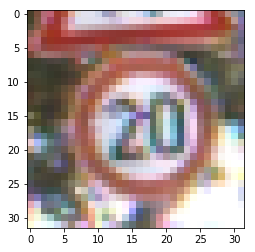The next step would be to declare some settings and convert the images to data types which are compatible with tensorflow:

``````# convert the data types
trainImages = trainImages.astype(np.float16)
trainLabels = trainLabels.astype(np.int32)

# Basic settings
NUM_CLASSES = 43
BATCH_SIZE = 16
N_TRAINING_DATA = trainImages.shape
EPOCHS = 2
# using python3 ;)
TRAINING_STEPS = N_TRAINING_DATA // BATCH_SIZE
``````

The following histogram shows the size of the images and justifies the opinion why scaling all images to 32x32 pixels could be alright.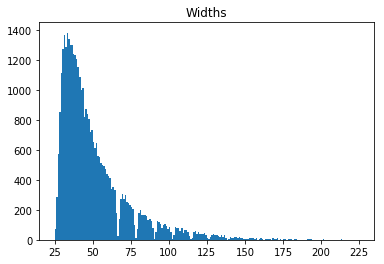## The first model

My first model was my (the starter) CIFAR-10 CNN. It consisted of two convolutional layers with max-pooling, a dense layer and softmax in the end. The input images were of size 32x32x3 and SGD was used to minimize the loss.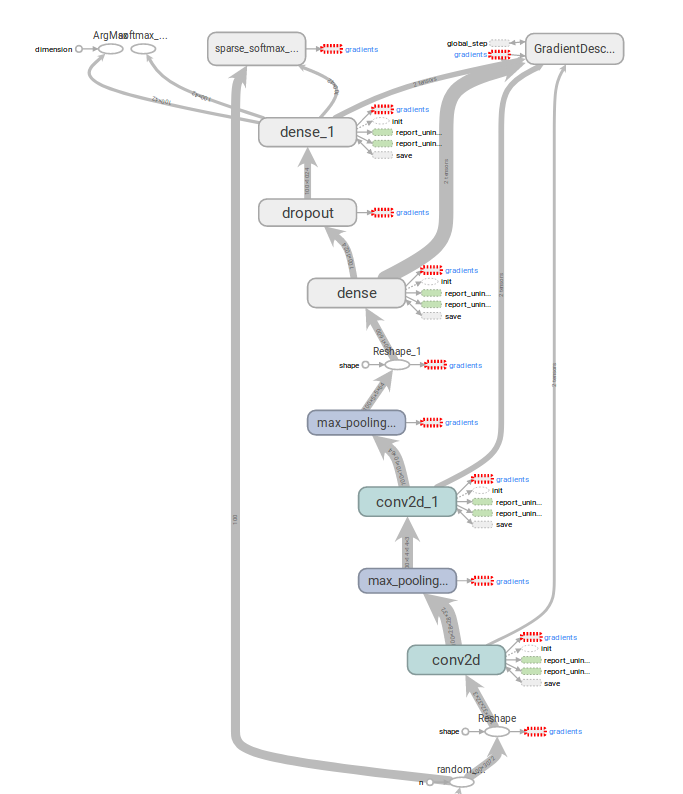After training for an hour or two the learning curve got too flat so I stopped the training process.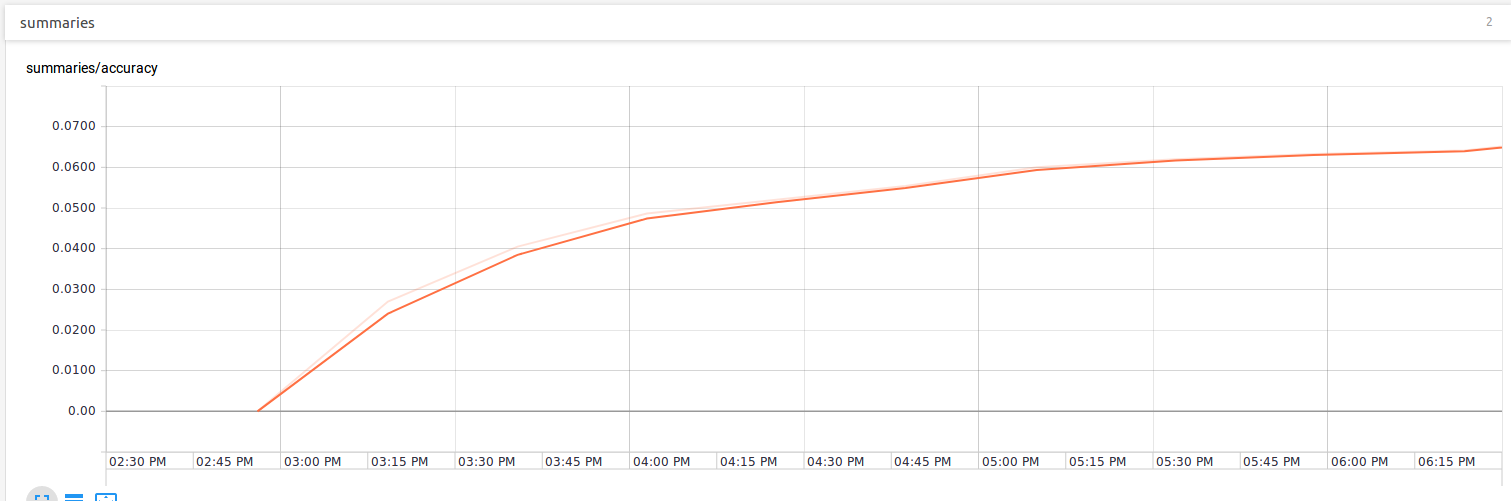## The second model

My first guess was that the model just was not capable enough so I took a look at VGG net, reduced the size of the conv filter to 3x3 and made the model deeper (4 convolutional layers with more filters, image size 64x64, an additional dense layer with 2048 units.

It felt like crypto mining on my laptop. Every step of that network took more than 20 seconds but it was late so I decided to stop for the day, dim the brightness and let the network train…

72 hours later! :D

So I came back, refreshed the Tensorboard and realized, that the model was not at the accuracy at which the smaller model was after 1 hour of training.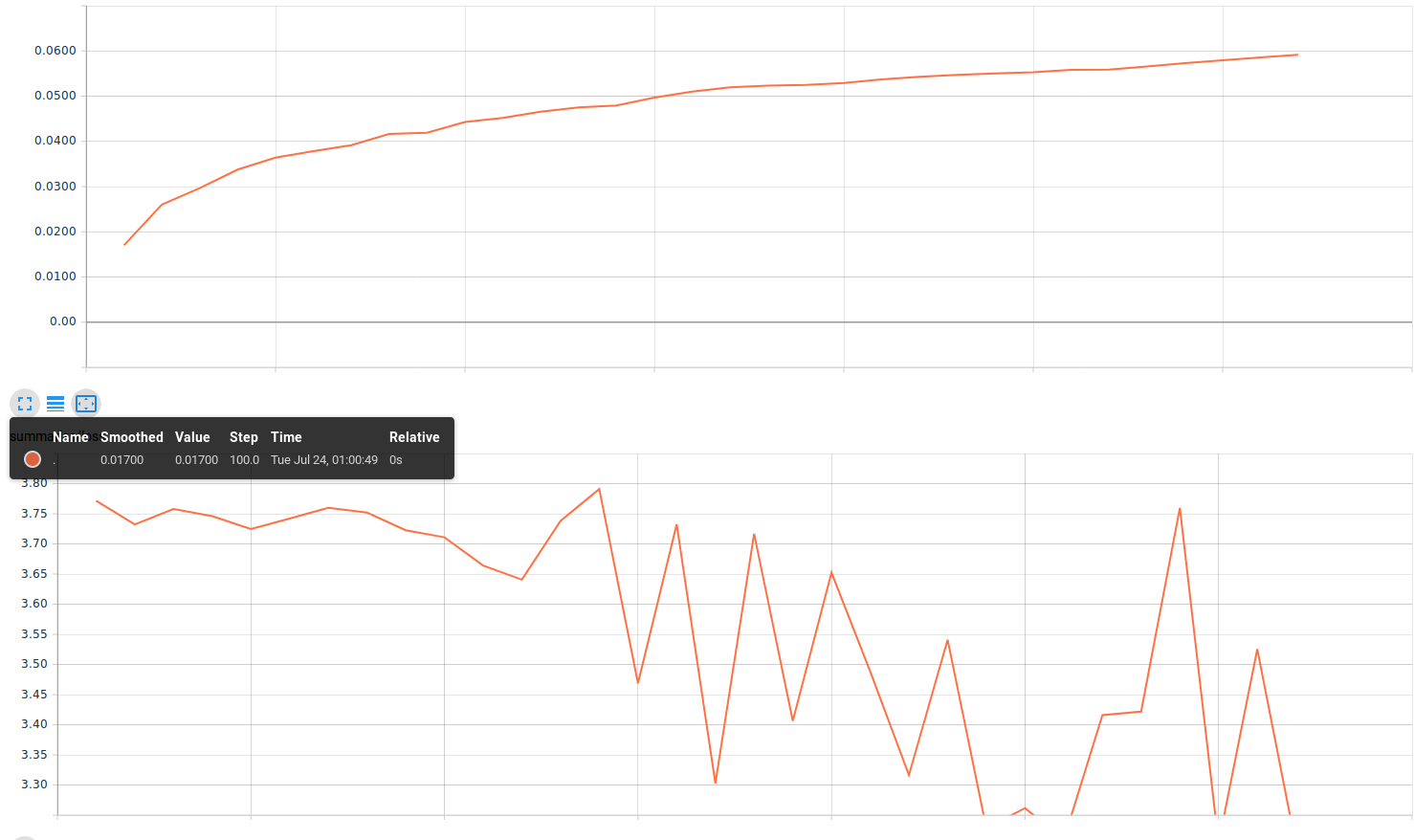I stopped the training… Although my hardware is really limited and I have to do something about this, my model has to be the primary problem. But how should I know what’s wrong if I can’t “see” into the model.

## Adding more summaries to the Tensorboard

Adding summaries is not as easy as I thought because of some reasons:

1. I am using tensorflow’s higher level `tf.layers` API so it is not straight forward to get the variables I am looking for
2. What variables should I plot? What is meaningful? Is it already a problem if the loss does not go down or should I plot the L2 norm of the weights?
3. How to visualize the convolutional filters?

I imagined there to be some easy to use builtin methods or just something like “… tf.layers.conv2d(visualize=True…)” option but that is not the case.

My first conv layer ended up looking like this:

``````# Convolutional Layer #1
conv1 = tf.layers.conv2d(
inputs=input_layer,
filters=32,
kernel_size=[3, 3],
activation=tf.nn.relu,
name='conv1'
)

with tf.variable_scope('conv1', reuse=tf.AUTO_REUSE) as scope_conv:
weights = tf.get_variable('kernel', dtype=tf.float16)

# scale weights to [0 255] and convert to uint8 (maybe change scaling?)
x_min = tf.reduce_min(weights)
x_max = tf.reduce_max(weights)
weights_0_to_1 = (weights - x_min) / (x_max - x_min)
weights_0_to_255_uint8 = tf.image.convert_image_dtype (weights_0_to_1, dtype=tf.uint8)

# to tf.image_summary format [batch_size, height, width, channels]
weights_transposed = tf.transpose (weights_0_to_255_uint8, [3, 0, 1, 2])

# this will display random 3 filters from the 64 in conv1
tf.summary.image('conv1/filters', weights_transposed, max_outputs=3)
``````

## The third model

I reduced the complexity of the model and introduced Adam in order to minimize the cross_entropy. Let’s see whether that helps. If not I have got some more summaries to look into…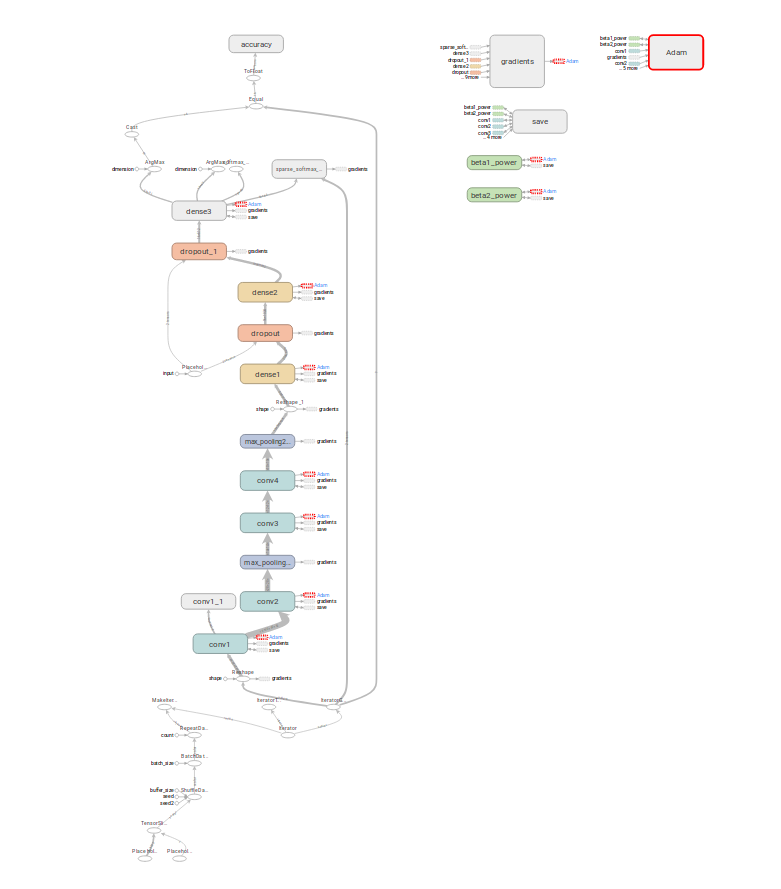The weight visualization looks suspicious to me. In the first run every filter is really colorful and after that everything is dead? This behaviour is not really probable.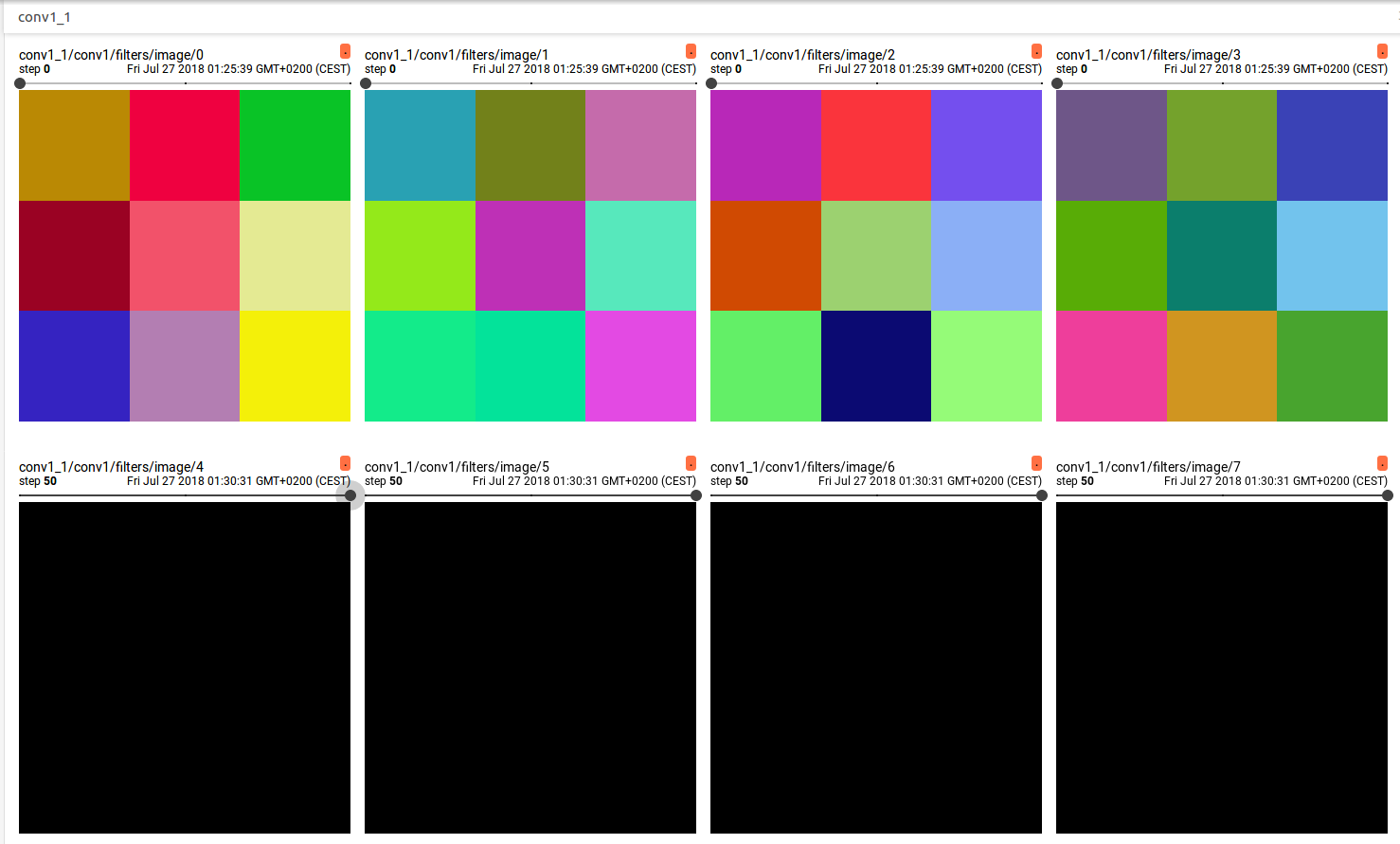The accuracy is 0.005 right now. I am going to wait whether something is going to happen here. Okay, I see that if every filter is black that the network cannot learn anything though. Maybe Adam has to be initialized in a special way…

But for right now I am switching to SGD with momentum with a large learning rate: `optimizer = tf.train.MomentumOptimizer(0.01, 0.9)`

After 4 hours of training the accuracy was at 65% and the curve still pointed up slightly.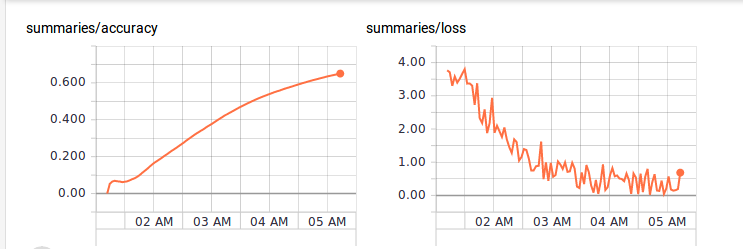## Data set augmentation

I wanted to try data set augmentation. What a coincidence that I started with randomly flipping images and realize that that’s a bad idea with traffic signs because if you mirror some of them along a vertical axis you also change the meaning. As it is visible in the following image: Traffic signs are not symmetric per se.